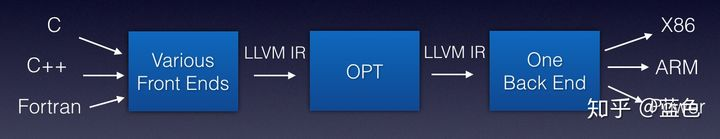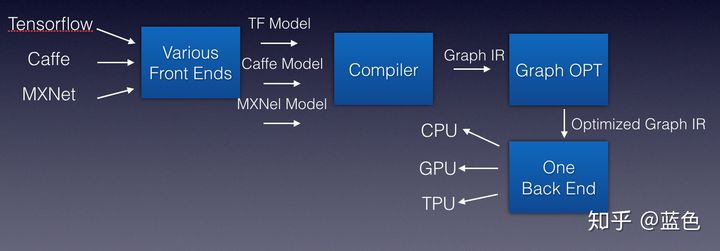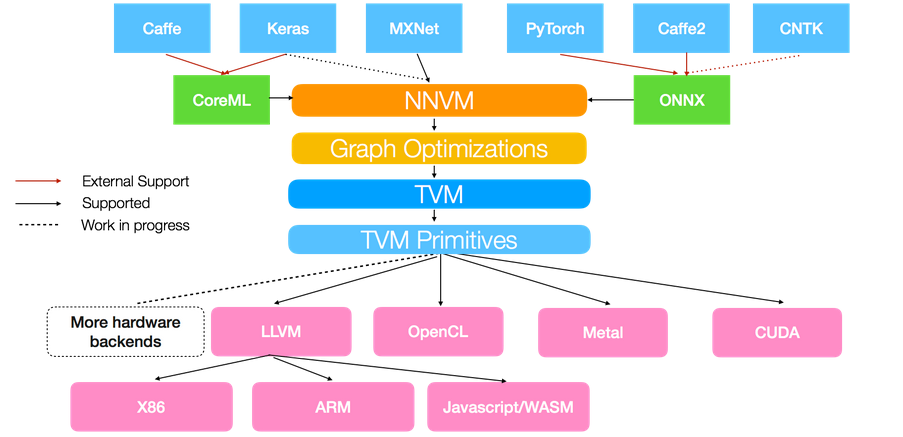# 0x1. 为什么需要深度学习编译器？¶

• 编译器前端：接收C/C++/Java等不同语言，进行代码生成，吐出IR
• 编译器中端：接收IR，进行不同编译器后端可以共享的优化，如常量替换，死代码消除，循环优化等，吐出优化后的IR
• 编译器后端：接收优化后的IR，进行不同硬件的平台相关优化与硬件指令生成，吐出目标文件# 0x02. TVM¶# 0x03. 环境配置¶

## 0x03.1 基于Docker的方式¶

docker pull tvmai/demo-gpu
nvidia-docker run --rm -it tvmai/demo-gpu bash


## 0x03.2 本地编译以Ubuntu为例¶

git clone --recursive https://github.com/apache/tvm tvm
cd tvm
mkdir build
cp cmake/config.cmake build
cd build
cmake ..
make -j4
export TVM_HOME=/path/to/tvm
export PYTHONPATH=$TVM_HOME/python:${PYTHONPATH}


# 0x04. 样例展示¶

Relay top-1 id: 282, class name: tiger cat
Torch top-1 id: 282, class name: tiger cat
Relay time:  1.1846002000000027 seconds
Torch time:  2.4181047000000007 seconds


## 0x04.1 导入TVM和Pytorch并加载ResNet18模型¶

import time
import tvm
from tvm import relay

import numpy as np

# PyTorch imports
import torch
import torchvision

######################################################################
# Load a pretrained PyTorch model
# -------------------------------
model_name = "resnet18"
model = getattr(torchvision.models, model_name)(pretrained=True)
model = model.eval()

# We grab the TorchScripted model via tracing
input_shape = [1, 3, 224, 224]
input_data = torch.randn(input_shape)
scripted_model = torch.jit.trace(model, input_data).eval()


## 0x04.2 载入测试图片¶

from PIL import Image

img_url = "https://github.com/dmlc/mxnet.js/blob/main/data/cat.png?raw=true"
img = Image.open(img_path).resize((224, 224))

# Preprocess the image and convert to tensor
from torchvision import transforms

my_preprocess = transforms.Compose(
[
transforms.Resize(256),
transforms.CenterCrop(224),
transforms.ToTensor(),
transforms.Normalize(mean=[0.485, 0.456, 0.406], std=[0.229, 0.224, 0.225]),
]
)
img = my_preprocess(img)
# 新增Batch维度
img = np.expand_dims(img, 0)


## 0x04.3 Relay导入TorchScript模型并编译到LLVM后端¶

######################################################################
# Import the graph to Relay
# -------------------------
# Convert PyTorch graph to Relay graph. The input name can be arbitrary.
input_name = "input0"
shape_list = [(input_name, img.shape)]
mod, params = relay.frontend.from_pytorch(scripted_model, shape_list)

######################################################################
# Relay Build
# -----------
# Compile the graph to llvm target with given input specification.
target = "llvm"
target_host = "llvm"
ctx = tvm.cpu(0)
with tvm.transform.PassContext(opt_level=3):
lib = relay.build(mod, target=target, target_host=target_host, params=params)


## 0x04.4 在目标硬件上进行推理并输出分类结果¶

######################################################################
# Execute the portable graph on TVM
# ---------------------------------
# Now we can try deploying the compiled model on target.
from tvm.contrib import graph_runtime

tvm_t0 = time.clock()
for i in range(10):
dtype = "float32"
m = graph_runtime.GraphModule(lib["default"](ctx))
# Set inputs
m.set_input(input_name, tvm.nd.array(img.astype(dtype)))
# Execute
m.run()
# Get outputs
tvm_output = m.get_output(0)
tvm_t1 = time.clock()


#####################################################################
# Look up synset name
# -------------------
# Look up prediction top 1 index in 1000 class synset.
synset_url = "".join(
[
"pretrained-models.pytorch/master/data/",
"imagenet_synsets.txt",
]
)
synset_name = "imagenet_synsets.txt"
with open(synset_path) as f:

synsets = [x.strip() for x in synsets]
splits = [line.split(" ") for line in synsets]
key_to_classname = {spl: " ".join(spl[1:]) for spl in splits}

class_url = "".join(
[
"pretrained-models.pytorch/master/data/",
"imagenet_classes.txt",
]
)
class_name = "imagenet_classes.txt"
with open(class_path) as f:

class_id_to_key = [x.strip() for x in class_id_to_key]

# Get top-1 result for TVM
top1_tvm = np.argmax(tvm_output.asnumpy())
tvm_class_key = class_id_to_key[top1_tvm]

# Convert input to PyTorch variable and get PyTorch result for comparison
torch_t0 = time.clock()
for i in range(10):
torch_img = torch.from_numpy(img)
output = model(torch_img)

# Get top-1 result for PyTorch
top1_torch = np.argmax(output.numpy())
torch_class_key = class_id_to_key[top1_torch]
torch_t1 = time.clock()

tvm_time = tvm_t1 - tvm_t0
torch_time = torch_t1 - torch_t0

print("Relay top-1 id: {}, class name: {}".format(top1_tvm, key_to_classname[tvm_class_key]))
print("Torch top-1 id: {}, class name: {}".format(top1_torch, key_to_classname[torch_class_key]))
print('Relay time: ', tvm_time / 10.0, 'seconds')
print('Torch time: ', torch_time / 10.0, 'seconds')


# 0x06. 参考¶